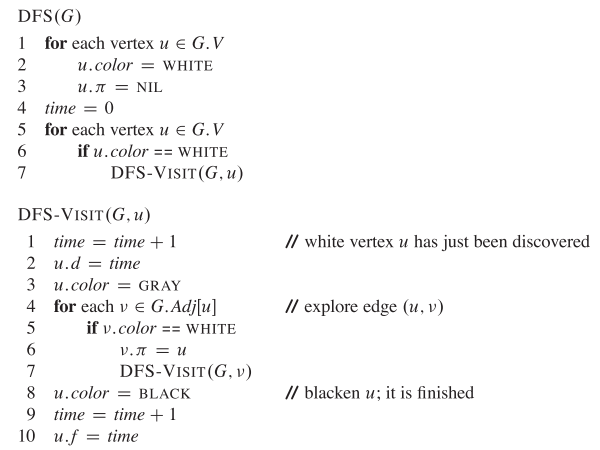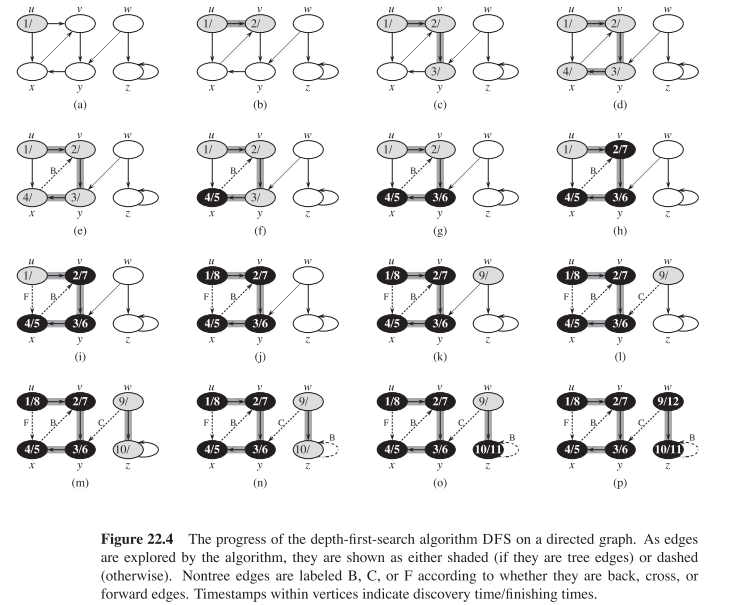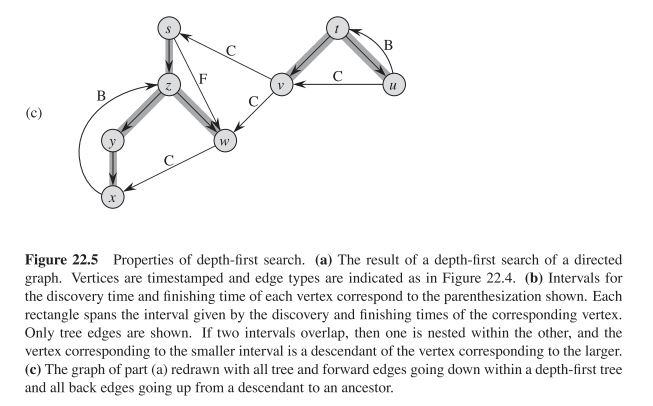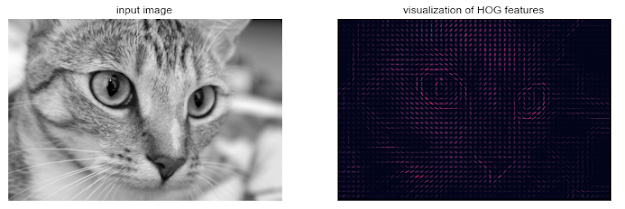## 2013年4月9日 星期二

### [ Intro Alg ] Elementary Graph Algorithms - Depth-ﬁrst search

Depth-ﬁrst search:
The strategy followed by depth-ﬁrst search is, as its name implies, to search “deeper” in the graph whenever possible. Depth-ﬁrst search explores edges out of the most recently discovered vertex that still has unexplored edges leaving it. Once all of ’s edges have been explored, the search “backtracks” to explore edges leaving the vertex from which was discovered. This process continues until we have discovered all the vertices that are reachable from the original source vertex. If any undiscovered vertices remain, then depth-ﬁrst search selects one of them as a new source, and it repeats the search from that source. The algorithm repeats this entire process until it has discovered every vertex.

As in breadth-ﬁrst search, whenever depth-ﬁrst search discovers a vertex during a scan of the adjacency list of an already discovered vertex u, it records this event by setting ’s predecessor attribute : to u. Unlike breadth-ﬁrst search, whose predecessor subgraph forms a tree, the predecessor subgraph produced by a depth-ﬁrst search may be composed of several trees, because the search may repeat from multiple sources. Therefore, we deﬁne the predecessor subgraph of a depth-ﬁrst search slightly differently from that of a breadth-ﬁrst search:The predecessor subgraph of a depth-ﬁrst search forms a depth-ﬁrst forest comprising several depth-ﬁrst trees. As in breadth-ﬁrst search, depth-ﬁrst search colors vertices during the search to indicate their state. Each vertex is initially white, is grayed when it is discovered in the search, and is blackened when it is ﬁnished, when its adjacency list has been examined completely. This technique guarantees that each vertex ends up in exactly one depth-ﬁrst tree, so that these trees are disjoint.

Besides creating a depth-ﬁrst forest, depth-ﬁrst search also timestamps each vertex. Each vertex v has two timestamps: the ﬁrst timestamp v.d records when is ﬁrst discovered (and grayed), and the second timestamp v.f records when the search ﬁnishes examining ’s adjacency list (and blackens v). These timestamps provide important information about the structure of the graph and are generally helpful in reasoning about the behavior of depth-ﬁrst search.

The procedure DFS below records when it discovers vertex u in the attribute u.d and when it ﬁnishes vertex u in the attribute u.f . These timestamps are integers between 1 and 2 |V|, since there is one discovery event and one ﬁnishing event for each of the |V| vertices. For every vertex u. The following pseudocode is the basic depth-ﬁrst-search algorithm. The input graph G may be undirected or directed. The variable time is a global variable that we use for timestamping.Below figure illustrates the progress of DFSThe running time of DFS is θ(V + E)

Properties of depth-ﬁrst search:
Depth-ﬁrst search yields valuable information about the structure of a graph. Perhaps the most basic property of depth-ﬁrst search is that the predecessor sub-graph G does indeed form a forest of trees, since the structure of the depth-ﬁrst trees exactly mirrors the structure of recursive calls of DFS-VISIT. That is, u=v.π if and only ifDFS-VISIT(G,v) was called during a search of u’s adjacency list. Additionally, vertex v is a descendant of vertex u in the depth-ﬁrst forest if and only if v is discovered during the time in which u is gray.

Another important property of depth-ﬁrst search is that discovery and ﬁnishing times have parenthesis structure. If we represent the discovery of vertex u with a left parenthesis “(u” and represent its ﬁnishing by a right parenthesis “u)”, then the history of discoveries and ﬁnishings makes a well-formed expression in the sense that the parentheses are properly nested. Below figures are examples:Java Implementation:

- enum EColor: 標示 Vertex 的顏色
1. package intralg.ch22;
2.
3. public enum EColor {
4.     White("White"),Gray("Gray"),Black("Black");
5.     private String msg;
6.     private EColor(String msg){this.msg = msg;}
7.     @Override
8.     public String toString(){return msg;}
9. }
- class Vertex: 代表 Graph 中的 Vertex, 從屬性 adjacent_list 可以知道該點的鄰近點.
1. package intralg.ch22;
2.
4.
5. public class Vertex {
6.
8.     public E data; // data
9.     public int d=Integer.MAX_VALUE; // distance or timestamp in DFS
10.     public int f=Integer.MAX_VALUE; // timestamp in DFS
11.     public EColor color=EColor.White; // White/Gray/Black
12.     public Vertex pi; // parent, predecessor
15.     {
17.     }
18.
19.     @Override
20.     public String toString()
21.     {
22.         //return String.format("{data:%s,distance:%d,color:%s,pi:%s}", data, d, color, pi!=null?pi.data:"NIL");
23.         return String.format("{data:%s,d:%d,f:%d,color:%s,pi:%s}", data, d, f, color, pi!=null?pi.data:"NIL");
24.     }
25. }
- class GAlg: 在這個類別我們定義一些常用的 Graph algorithms, 這邊會用到的是 DFS
1. package intralg.ch22;
2.
3. import java.util.ArrayList;
5. import java.util.List;
6. import java.util.Queue;
7.
8. public class GAlg {
9.     @SuppressWarnings("unchecked")
10.     public static List> GenDFSData2()
11.     {
12.         List> vertex_list = new ArrayList>();
13.         Vertex s = new Vertex('s'); vertex_list.add(s);
14.         Vertex t = new Vertex('t'); vertex_list.add(t);
15.         Vertex u = new Vertex('u'); vertex_list.add(u);
16.         Vertex v = new Vertex('v'); vertex_list.add(v);
17.         Vertex w = new Vertex('w'); vertex_list.add(w);
18.         Vertex x = new Vertex('x'); vertex_list.add(x);
19.         Vertex y = new Vertex('y'); vertex_list.add(y);
20.         Vertex z = new Vertex('z'); vertex_list.add(z);
29.         return vertex_list;
30.     }
31.     @SuppressWarnings("unchecked")
32.     public static List> GenDFSData()
33.     {
34.         List> vertex_list = new ArrayList>();
35.         Vertex u = new Vertex('u'); vertex_list.add(u);
36.         Vertex v = new Vertex('v'); vertex_list.add(v);
37.         Vertex w = new Vertex('w'); vertex_list.add(w);
38.         Vertex x = new Vertex('x'); vertex_list.add(x);
39.         Vertex y = new Vertex('y'); vertex_list.add(y);
40.         Vertex z = new Vertex('z'); vertex_list.add(z);
47.         return vertex_list;
48.     }
49.
50.
51.     public static int TIME = 0;
52.     public static void _DFS_VISIT(Vertex u)
53.     {
54.         TIME += 1;
55.         u.d = TIME;
56.         u.color = EColor.Gray;
57.         System.out.printf("\t\tDiscover %s...\n", u);
59.         {
60.             if(v.color.equals(EColor.White))
61.             {
62.                 _DFS_VISIT(v);
63.                 v.pi = u;
64.             }
65.         }
66.         u.color = EColor.Black;
67.         TIME += 1;
68.         u.f = TIME;
69.         System.out.printf("\t[Info] Vertex=%s is visited!\n", u);
70.     }
71.
72.     public static void DFS(List> graph)
73.     {
74.         // Initialize
75.         TIME = 0;
76.         for(Vertex v:graph)
77.         {
78.             v.pi = null;
79.             v.d = v.f = 0;
80.             v.color = EColor.White;
81.         }
82.
83.         for(Vertex v:graph)
84.         {
85.             if(v.color.equals(EColor.White)) _DFS_VISIT(v);
86.         }
87.
88.         /*for(Vertex v:graph)
89.         {
90.             if(v.color.equals(EColor.White)) System.out.printf("\t[Info] Vertex=%s is not visited!\n", v);
91.         }*/
92.     }
93.
94.
95.     public static void main(String args[])
96.     {
97.         List> graph = GenDFSData2();
98.         DFS(graph);
99.     }
100. }

Discover {data:s,d:1,f:0,color:Gray,pi:NIL}...
Discover {data:z,d:2,f:0,color:Gray,pi:NIL}...
Discover {data:y,d:3,f:0,color:Gray,pi:NIL}...
Discover {data:x,d:4,f:0,color:Gray,pi:NIL}...
[Info] Vertex={data:x,d:4,f:5,color:Black,pi:NIL} is visited!
[Info] Vertex={data:y,d:3,f:6,color:Black,pi:NIL} is visited!
Discover {data:w,d:7,f:0,color:Gray,pi:NIL}...
[Info] Vertex={data:w,d:7,f:8,color:Black,pi:NIL} is visited!
[Info] Vertex={data:z,d:2,f:9,color:Black,pi:NIL} is visited!
[Info] Vertex={data:s,d:1,f:10,color:Black,pi:NIL} is visited!
Discover {data:t,d:11,f:0,color:Gray,pi:NIL}...
Discover {data:v,d:12,f:0,color:Gray,pi:NIL}...
[Info] Vertex={data:v,d:12,f:13,color:Black,pi:NIL} is visited!
Discover {data:u,d:14,f:0,color:Gray,pi:NIL}...
[Info] Vertex={data:u,d:14,f:15,color:Black,pi:NIL} is visited!
[Info] Vertex={data:t,d:11,f:16,color:Black,pi:NIL} is visited!

### [Py DS] Ch5 - Machine Learning (Part14)

Application: A Face Detection Pipeline This chapter has explored a number of the central concepts and algorithms of machine learning. But mo...